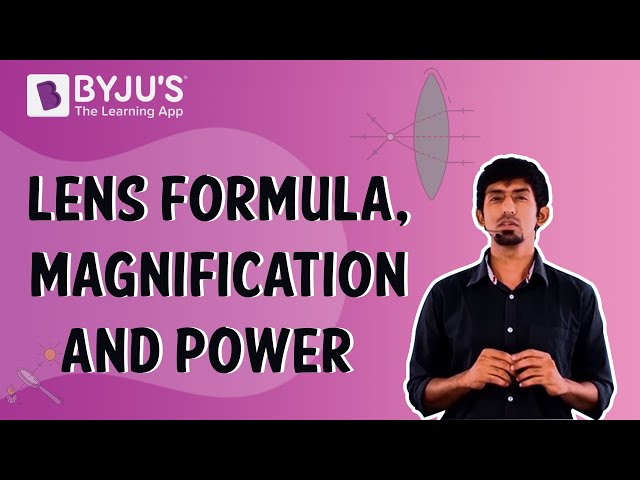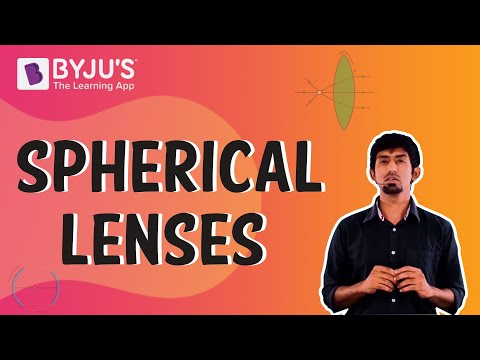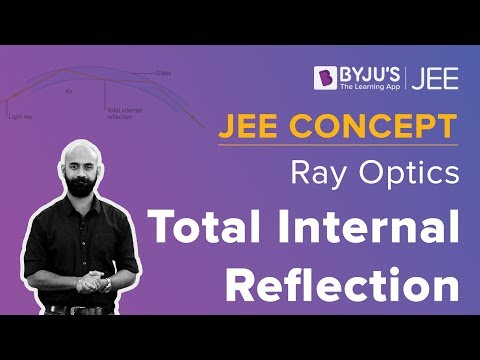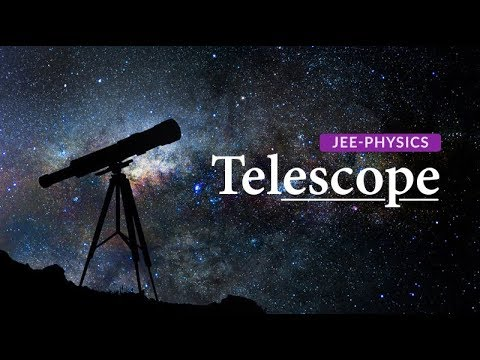# CBSE Class 12 Physics Notes Chapter 9 Ray Optics and Optical Instruments

The laws of refraction and reflection are valid for all the surfaces and pairs of media at the point of incidence.

## How Is Image Formed by a Lens?

The real image of a body which is being positioned between the 2f and f from a convex lens can be captured on a screen which has been placed at the location of the image even if the screen is removed. The screen simply diffuses the rays from a given point on the object, converging to an image in space and again diverging away. The laser show projection is an appropriate example of this phenomenon.The formation of an image needs regular refraction/reflection. The rays generated from a single point are expected to reach the same image point. That is why certain types of objects, such as the page of a book, don’t produce an image.

### What Are the Constituents of White Light?

The variety of colours of objects is witnessed due to the presence of constituents colours of the light falling upon them. White light may produce a different perception of the colours of a substance when compared with monochromatic light. Thick lenses also produce coloured images due to dispersion.

In the case of a simple microscope, the angular size of the object is similar to the angular size of the image. Still, it produces magnified results because the position of the object is closer to the eye, and hence the subtended angle will be larger.

The human eye is a convex lens having a focal length of 2.5 cm. The image is always formed in the retina and this ability of the eye is termed as accommodation.

### What Are the Uses of the Lens?

In the case of myopia, the image is focused before the retina, which can be fixed with the help of a diverging lens. In the case of hypermetropia, the image is focused beyond the retina, which can be fixed with the help of a converging lens. Cylindrical type of lenses is used to fix astigmatism.

#### For more information on Spherical Lenses and Total Internal Reflection, watch the below videos### What Is the Magnifying Power of a Simple Microscope?

The formula for finding the magnifying power of a single microscope is m=1 + (D/f). The distinct vision has the least distance of 25 cm. If the image is at infinity and f is referred to as the focal length, then m=D/f.

### What Is the Magnifying Power of a Compound Microscope?

The formula for the magnifying power is m=

$$\begin{array}{l}m_{e} * m_{o}\end{array}$$
in which
$$\begin{array}{l}m_{e}= 1+(D/f_{e})\end{array}$$
is termed as the eyepiece magnification and
$$\begin{array}{l}m_{o}\end{array}$$
is the objective lens magnification.

#### To know about Telescope, watch the below videoTo know more about ray optics and other related topics, keep visiting BYJU’S website.

### Important Questions

1. The size of a small candle is 4.5 cm and it is placed 30 cm in front of a concave mirror with a radius of curvature 40 cm. In order to obtain a sharp image, the distance from the mirror where the screen should be placed is? If the candle is moved closer to the mirror, how would the screen have to be moved and Describe the nature and size of the image.
2. In front of a convex mirror of focal length 20 cm, a needle of 5 cm is placed at a distance of 15 cm from it. Find out what happens as the needle is moved farther from the mirror. Find out the location of the image and its magnification.
3. What is the refractive index of water when a tank is filled with water to a height of 15 cm? The apparent depth of a needle lying at the bottom of the tank is 10 cm. If water is replaced by a liquid of refractive index 2 up to the same height, by what distance would the microscope have to be moved to focus on the needle again?

Related Topics:

NCERT Solutions for Ray Optics

NCERT Exemplars for Ray Optics

Ray Optics Important Questions

What is Optics

## Frequently Asked Questions on CBSE Class 12 Physics Notes Chapter 9 Ray Optics and Optical Instruments

Q1

### What is Len’s formula?

According to the Convex Lens equation, the Lens formula is 1/f = 1/v + 1/u. It relates the Focal Length of a Lens with the distance of an object which is placed in front of it and the image formed of that object.

Q2

### What are the uses of a telescope?

There are three basic functions of a telescope: light gathering, resolving and magnifying.

Q3

### What is ray optics?

Geometrical optics, or ray optics, is a model of optics that describes light propagation in terms of rays.